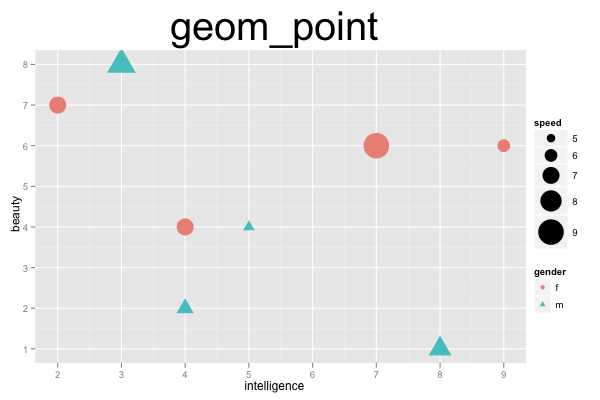## ggplot2 Quick Reference: geom_point

A geom that draws a point defined by an x and y coordinate.

Default statistic: stat_identity

### Parameters

• x - (required) x coordinate of the point
• y - (required) y coordinate of the point
• size - (default: 0.5) diameter of the point
• shape - (default: 16=dot) the shape of the point
• colour - (default: "black") the color of the point
• fill - (default: NA) the fill of the point (only a small minority of shapes actually can be filled; see shape)
• alpha - (default: 1=opaque) the transparency of the point
• na.rm - (default: FALSE) silently remove points with NA coordinates

### Example

This example shows a scatterplot. It represents a rather common configuration (just a `geom_point` layer with use of some extra aesthetic parameters, such as size, shape, and color). The plot uses two aesthetic properties to represent the same aspect of the data (the `gender` column is mapped into a shape and into a color), which is possible but might be a bit overdone. The plot maps the continuous `speed` column onto the aesthetic `size` property. To ensure that even observations with a "low" speed are still mapped to rather large points, the plot explicitly uses `scale_size_continuous` to define the range of point sizes to use.```d=data.frame(beauty=c(1,2,6,4,4,6,7,8), intelligence=c(8,4,7,5,4,9,2,3), speed=c(7,6,9,5,7,6,7,8), gender=c('m','m','f','m','f','f','f','m'))
ggplot() +
scale_size_continuous(to=c(4,12)) +
geom_point(data=d, mapping=aes(x=intelligence, y=beauty, shape=gender, color=gender, size=speed)) +
opts(title="geom_point", plot.title=theme_text(size=40, vjust=1.5))```